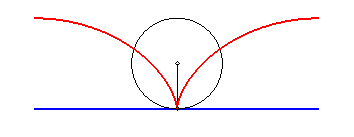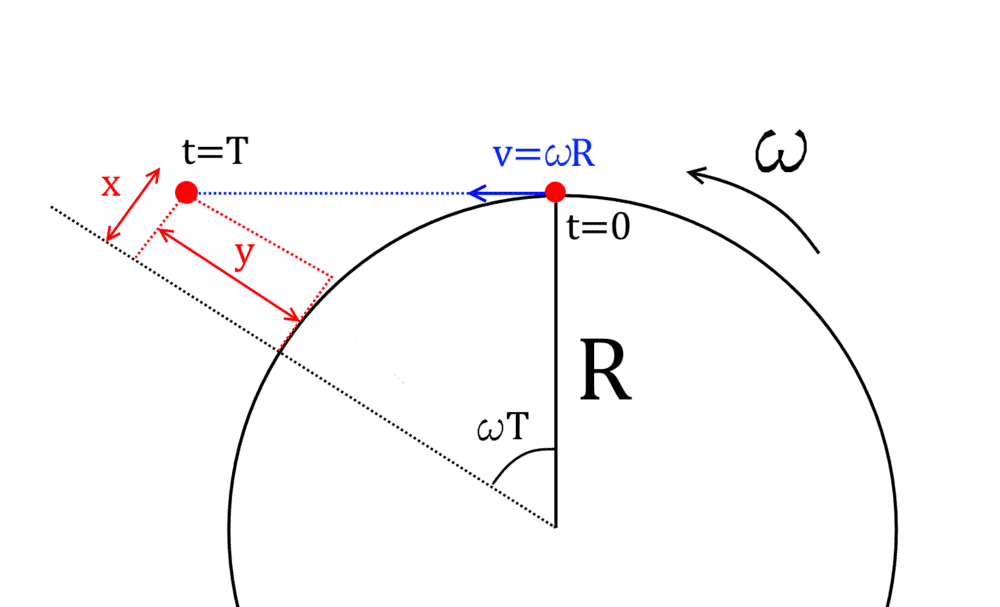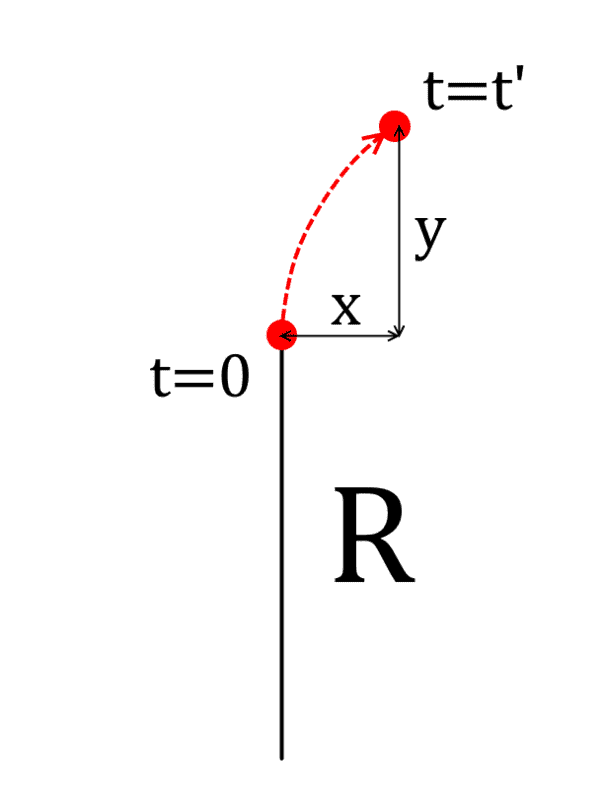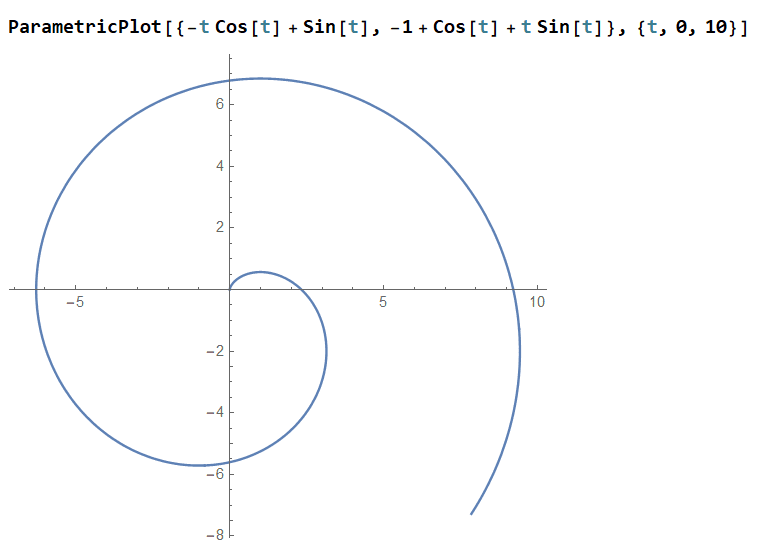# Rectilinear movement seen from a rotating reference frame

• Livio Arshavin Leiva
In summary: Please, see:https://en.wikipedia.org/wiki/Cycloidhttps://mathcurve.com/courbes2d.gb/cycloid/cycloid.shtmlYes, you can do the problem in terms of fictitious forces assuming that the release object follows a cycloid trajectory in the rotating frame.

#### Livio Arshavin Leiva

Let's suppose there's some platform that is rotating with angular speed omega and has a radius R. At t=0 we release some object from the border, which has an initial speed perpendicular to the radius direction with magnitude $$\omega R$$ and we want to know its position at t=T with respect to the initial point in the rotating frame, shown below with the coordinates {x,y}. We know the object will move along a tangent trajectory, shown with blue dot line. The length of the blue dot line is $$\omega R T$$ which is the same length of the arc covered in time T.
https://www.physicsforums.com/attachments/266688
Since this is just a geometry problem, after some calculations I reach the following results:
$$x=R(\sin(\omega T)-\omega T \cos(\omega T))$$
$$y=R(\cos(\omega T)+\omega T \sin(\omega T))-R$$
And it looks fine. I can also differentiate two times with respect to T to get the accelerations:
$$a_x=R\omega^2(\sin(\omega T)+\omega T \cos(\omega T))$$
$$a_y=R\omega^2(\cos(\omega T)-\omega T \sin(\omega T))$$
Ok, I'm quite convinced those expressions are right.

But now I imagine the problem from the rotating frame point of view. The trajectory of the released object would look more or less like the red dot arrow I think, as shown in the image below:
https://www.physicsforums.com/attachments/266685
Since no real force is acting on the object, in this case I have to explain the movement of the object in terms of two fictitious forces: the centrifugal force, responsible of movement along y direction, and the Coriolis force responsible of the movement along x direction, I think. For a 2D problem, we can write the acceleration as:
$$\vec a=\vec a_r+\vec a_\theta= (\ddot r-\dot\theta^2r)\hat r + (r \ddot \theta + 2 \dot \theta \dot r) \hat \theta$$
But here I don't understand how to introduce the angular speed of the rotating frame, so I've not succeeded to formulate the problem in terms of the fictitious forces to reach the previous result. If any of you knows, I would be very thankful if you can show me.

For some reason, I can't see the attached diagrams.

Livio Arshavin Leiva said:
...
But here I don't understand how to introduce the angular speed of the rotating frame, so I've not succeeded to formulate the problem in terms of the fictitious forces to reach the previous result. If any of you knows, I would be very thankful if you can show me.
I am unable to help you with the math of this problem, but it seems to me that the solution may be equivalent to the equation of motion for the cycloid trajectory of a point of a wheel (the point of reference in the edge of the platform in your problem) that is rolling over a horizontal surface (the rectilinear trajectory of the released object in your problem, which rotates with ω velocity respect to that point of reference).

https://en.wikipedia.org/wiki/Cycloid

https://mathcurve.com/courbes2d.gb/cycloid/cycloid.shtmlLast edited:
•Livio Arshavin Leiva
Livio Arshavin Leiva said:
Ok, I'm quite convinced those expressions are right.
Are ##x## and ##y## Cartesian coordinates in the inertial frame? If so, I'm not convinced that your expressions are correct. Surely the trigonometric functions should depend on the release time (call this ##T_0##) while the linear velocity term depends on the time (you seem to have used ##T##). In fact, you can tell that your expressions aren't correct because the acceleration of an inertial particle expressed in inertial coordinates should be zero, which you clearly don't have.

In fact, without loss of generality, you could just write ##x=R\omega T##, ##y=-R## (give or take signs - I can't see your images so I'm assuming positive ##\omega## means anti-clockwise).

Lnewqban said:
For some reason, I can't see the attached diagrams.
I see... I don't know what happened. At first when I uploaded it looked fine... I spent several minutes making the figures. I will re upload as soon as I'm with that computer again.

Can someone tell me why I can not edit my own post anymore? Before there was an "edit" button that seems to be not available anymore. I would like to upload again the images that for some reason are not properly displayed anymore neither...
Any moderator can help me?

Ibix said:
In fact, without loss of generality, you could just write ##x=R\omega T##, ##y=-R## (give or take signs - I can't see your images so I'm assuming positive ##\omega## means anti-clockwise).These are the two images.

•Lnewqban
Ibix said:
Are ##x## and ##y## Cartesian coordinates in the inertial frame? If so, I'm not convinced that your expressions are correct. Surely the trigonometric functions should depend on the release time (call this ##T_0##) while the linear velocity term depends on the time (you seem to have used ##T##). In fact, you can tell that your expressions aren't correct because the acceleration of an inertial particle expressed in inertial coordinates should be zero, which you clearly don't have.Are the images visible now?
I know in the inertial frame the acceleration with respect to a fixed point should be zero...
But as you can see, x and y refer to a fixed point in the rotating frame.
I hope my question is more clear now... it's a pity the images are not displayed... but I can not edit the post anymore for some reason...

Lnewqban said:
I am unable to help you with the math of this problem, but it seems to me that the solution may be equivalent to the equation of motion for the cycloid trajectory of a point of a wheel (the point of reference in the edge of the platform in your problem) that is rolling over a horizontal surface (the rectilinear trajectory of the released object in your problem, which rotates with ω velocity respect to that point of reference).

https://en.wikipedia.org/wiki/Cycloid

https://mathcurve.com/courbes2d.gb/cycloid/cycloid.shtml

View attachment 266714
Very nice... indeed it seems to be an equivalent scenario. I'll read a little and then compare results.

•Lnewqban
Livio Arshavin Leiva said:
Can someone tell me why I can not edit my own post anymore? Before there was an "edit" button that seems to be not available anymore...
That "Edit" button is available only for certain amount of hours after the post is completed, then, it disappears.
Very nice schematics, thank you.

Clearly, the motion as seen will not be a cycloid. Although the initial behavior is very similar the eventual behavior as seen from the rotating frame will roughly approximate an archimedean spiral -- each successivel loop a fixed distance from the last.

The choice of a coordinate system which is both rotating and translating makes life difficult. Surely it would be simpler to shift to a coordinate system anchored to the center of rotation so that one has to contend with rotation alone?

Working from the rotating frame (and a coordinate system anchored to the center of rotation), my inclination would be to start with the centrifugal potential to derive the speed of the object at any given radius. Then it would be good if one had an analogue to conservation of angular momentum that works for the rotating frame. My intuition screams that there should be one.

Last edited:
•Livio Arshavin Leiva
So your question is how to write down the ##r,\theta## coordinates of the released particle in the rotating frame, so that you can feed the result into the equations for the inertial forces?

Write down the Cartesian coordinates of the particle in the inertial frame, transform this to polar coordinates, then subtract ##\omega t## from the polar coordinate to get rotating polars, taking care that your signs and origins are all consistent. Then feed it into the formulae you've got.

As a check, you could use ##r=\sqrt{x^2+(y+R)^2}## and ##\tan\theta=(y+R)/x## and feed that in. Again, some care about signs is needed for the angular coordinate.

jbriggs444 said:
Clearly, the motion as seen will not be a cycloid. Although the initial behavior is very similar the eventual behavior as seen from the rotating frame will roughly approximate an archimedean spiral -- each successivel loop a fixed distance from the last.

The choice of a coordinate system which is both rotating and translating makes life difficult. Surely it would be simpler to shift to a coordinate system anchored to the center of rotation so that one has to contend with rotation alone?

Working from the rotating frame (and a coordinate system anchored to the center of rotation), my inclination would be to start with the centrifugal potential to derive the speed of the object at any given radius. Then it would be good if one had an analogue to conservation of angular momentum that works for the rotating frame. My intuition screams that there should be one.
Actually I plotted the result I reached for R=1 and omega=1 and it looks like an spiral as you say:If I shift to a coordinate system anchored to the center of rotation I think the only difference is adding +R (in this case +1) in the "y" direction, isn't it?? I think formulating the centrifugal potential is not enough, since the particle is not only moving radially but also in theta direction, so a Coriolis acceleration should be considered too.

Livio Arshavin Leiva said:
Actually I plotted the result I reached for R=1 and omega=1 and it looks like an spiral as you say:
View attachment 266744
If I shift to a coordinate system anchored to the center of rotation I think the only difference is adding +R (in this case +1) in the "y" direction, isn't it?? I think formulating the centrifugal potential is not enough, since the particle is not only moving radially but also in theta direction, so a Coriolis acceleration should be considered too.
Yes, I agree that the choice of center amounts to offsetting coordinates by +1. So the center of the centrifugal potential will be at (0,-1) rather than at (0,0).

Yes, centrifugal potential is not enough. That just gives you speed. Coriolis acts at right angles to velocity, so it cannot affect speed. We would need another conservation principle if we are going to solve for direction as well as speed using the approach which I envision.

I think that the conserved quantity is ##\sum mv \times r - m\omega r^2## where ##\omega## is the rotation rate of the frame and ##r## is the vector distance from the center of rotation. Given energy as a function of distance from the center of rotation (the centrifugal force field), one could use this second conservation law to deduce tangential velocity. There should then be two equal and opposite radial velocities compatible with this tangential velocity -- one for the outward trajectory and one for the mirror-image inward trajectory. Unsurprisingly, this conserved quantity is simply angular momentum in the inertial frame transformed to the rotating frame. One might be able to invert the radial velocity to get ##\frac{dt}{dx}##, integrate over radial distance to get time as a function of radial distance and then invert again to get radial distance as a function of time.

Lots simpler to simply work in the inertial frame and then transform to the rotating frame.

Last edited:
•vanhees71
So let's simply do it. Without loss of generality we assume that the particle moves with constant velocity along the ##x## axis in the inertial frame, and we view the situation in a frame rotating with constant angular velocity around the ##z## axis. We need to calculate the coordinates ##(x,y)## in the inertial and ##(x',y')## coordinates in the rotating frame.

In the inertial frame it's easy:
$$\vec{r}(t)=(v t,0).$$
The transformation between the Cartesian coordinates of the inertial and the Cartesian coordinates of the inertial frame is
$$\begin{pmatrix} x' \\ y' \end{pmatrix} = \begin{pmatrix} \cos(\omega t) & \sin (\omega t) \\-\sin(\omega t) & \cos (\omega t) \end{pmatrix} \begin{pmatrix}x \\ y \end{pmatrix}.$$
For our uniformly moving point the coordinates are thus
$$\vec{r}'(t)=\begin{pmatrix} v t \cos(\omega t) \\ - v t \sin(\omega t) \end{pmatrix}.$$
Seen from the rotating frame, it's of course a spiral, as said in several previous posts already.

See the wiki on Coriolis Force for the formula for the fictional forces a body appears to experience when the motion is considered in a notating frame.

Why fictitious?

Because they are not real forces. If you want F=ma to apply in a rotating frame you have to invent other forces, dependent on the rotational speed, to get the body to move as it does move.

#### Attachments

Frodo said:
See the wiki on Coriolis Force for the formula for the fictional forces a body appears to experience when the motion is considered in a notating frame.

Why fictitious?

Because they are not real forces. If you want F=ma to apply in a rotating frame you have to invent other forces, dependent on the rotational speed, to get the body to move as it does move.

Yes, I checked those formulas and wrote the x and y components of the acceleration in the rotating frame, reaching the following system of entangled equations for x and y:
$$\ddot x = 2\omega\dot y + x\omega^2$$
$$\ddot y = -2\omega\dot x + y\omega^2$$
Honestly I don't remember exactly how to solve this manually but when I solved it with Wolfram Mathematica setting the boundary conditions for t=0 as x=0, y=R, vx=0 and vy=0, I got the same expressions in the original post. So I think it's verified now. This is what I wanted, to reach the same result by using the fictitious forces in the rotating frame. It's quite curious that a more or less complicated system of differential equations can be solved as a much more simple geometric problem if one if able to do the correct transformation of the coordinates. (In this case going back to the inertial frame)
Thank you all for your help.

•vanhees71 and Lnewqban